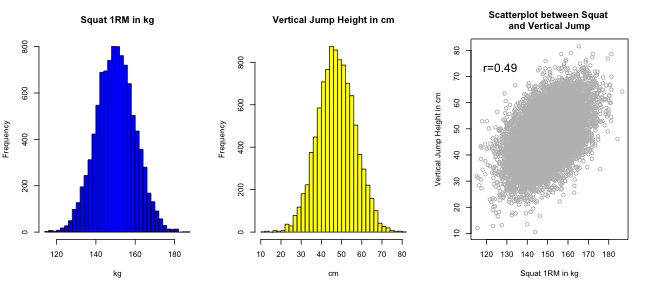Understanding Inferential Statistics Using Correlation Example

# Understanding Inferential Statistics Using Correlation Example

## Introduction

In the following R and knitr experiment/blog post I will be documenting my play with correlation and inferences. I am just reading Discovering Statistics Using R by Andy Field and I am trying to code some staff from the book, plus experiment and see how inferential statistics work.

Simulations are great way to learn statistics in my opinion and in opinion of Will Hopkins. I hope that someone might find this blog post interesting and learn a thing or two.

As I have pointed out in previous blog posts, sport coaches are not interested in inferential statistics, but rather individual reaction/effects, yet most if not all research utilize inferential statistics. Why is that? Because in research we are interested in effects overall (or on average) on a given population, and not on a single individual or sample. In research, subjects are just vehicles, a way to get numbers/estimates or observations, while in sport they are what matters the most.

Since it very hard to measure the whole population, we need to make inferences from smaller sample to the bigger population. To do this we use Central Limit Theorem and estimated standard error (it is beyond me why standard error is not called sampling error, because it conveys much more meaning).

Understanding this of crucial importance to understand statistics and I have struggled with this mainly because most books don't put much pages/emphasis on getting it and jump to ANOVAs and all thet fancy stuff too soon.

Enough of my rant – I hope that this blog post might yield some light on population/sample inferences for the students. I will use correlation as an estimate we are interested into (it could be mean, SD, Cohen's effect size, whatever – the idea is the same).

## Population correlation

Creating population with two estimates that correlate – in this case squat and vertical jump in athletes (NOTE: All data are imaginary for the sake of an example)

``````populationSize <- 10000

# Simulate vertical jump and squat estiamtes in population
randomError = 8
populationSquatKG <- rnorm(populationSize, mean = 150, sd = 10)
populationVerticalJumpCM <- populationSquatKG * 0.45 - 20 + rnorm(populationSize,
mean = 0, sd = randomError)

# Graph the populations and scatter
par(mfrow = c(1, 3))

hist(populationSquatKG, 30, col = "blue", xlab = "kg", main = "Squat 1RM in kg")

hist(populationVerticalJumpCM, 30, col = "yellow", xlab = "cm", main = "Vertical Jump Height in cm")

plot(populationSquatKG, populationVerticalJumpCM, col = "grey", main = "Scatterplot between Squat \nand Vertical Jump",
xlab = "Squat 1RM in kg", ylab = "Vertical Jump Height in cm")

# Add Text (r=) on the graph
text(min(populationSquatKG) * 1.1, max(populationVerticalJumpCM) * 0.9, paste("r=",
as.character(round(cor(populationSquatKG, populationVerticalJumpCM), 2)),
sep = ""), cex = 1.5)
``````In the population above r=0.49 between vertical jump and squat. Let's see what happens with correlation when we modify the random error parametemodify the random error parameter.Standard Membership
\$35 per month

OR